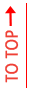# 5 Free Books to Learn Statistics for Data Science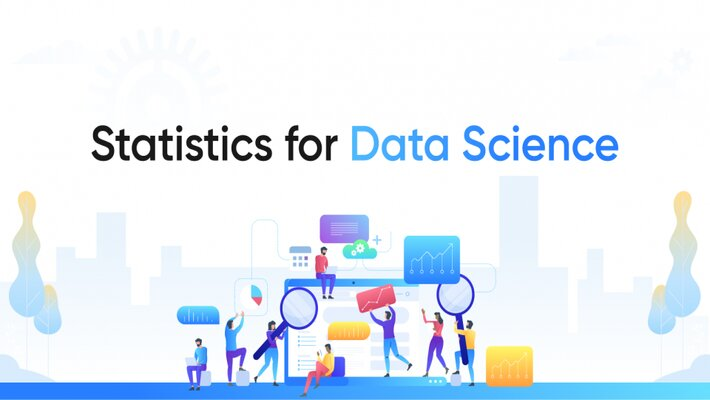“Facts are stubborn but statistics are more pliable quote mark twain” – Mark Twain

The immortal skill for data scientists, which is used every day, is statistics

Statistics allows data scientists to gather, define, understand, envisage, and infer data. Data scientists use statistics for data analysis, experiment design, and statistical modeling.

Beyond data science, machine learning is the field where statistics is implemented. Machine learning engineers use statistics for understanding the data before setting up a training model.

Professionals take data samples for training and testing purposes, for the models they input the data and statistical techniques for success probability.

Also, for the evaluation of the performance model, statistics is required for assessing the predictions' variability and accuracy measurement.

The above ways are just methods which are employed by data scientists.

If you are studying data science it is therefore essential to develop a good understanding of these statistical techniques.

In any field book reading is must. And statistics is no exception. It is one of the fields where books contribute largely as a tool for detailed explanations of statistical concepts, which are vital for understanding.

Here is a list of top 5 books for learning statistics for data science –

Peter and Andrew Bruce’s Practical Statistics for Data Scientists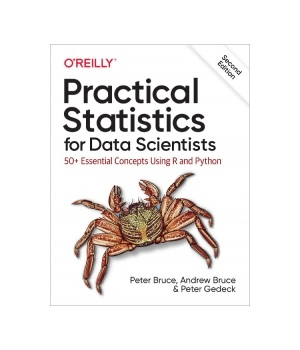This book includes major topics like data structures, descriptive statistics, probability, and machine learning, which are most suitable for beginners.

Statistics is a broad field, where only a portion of it is relevant to data science. It is a book which is exceptionally good for covering the fields of data science. Beginners can look up to this book and learn about data science practice.

This book covers related practical coded examples, written in R, which gives clear explanations for statistical terms used, and also links the resources for further reading.

It is an excellent book which covers the basics, and most suitable for an absolute beginner in the data science field.

Allen B. Downey’s Think Stats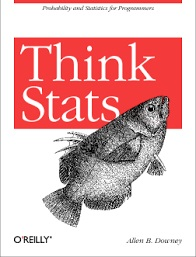This book includes major topics like statistical thinking, distributions, hypothesis testing, and correlation, which are most suitable for beginners with basic python.

In the beginning, the book states that “This book is about turning knowledge into data”, and it does maintain well with practical examples of data analysis.

It is another book which covers only the concepts directly applicable to data science, and also has a lot of coding examples which are written in Python. This is recommended for programmers, who are searching for understanding the key statistical concepts. It is suitable for those who have at least basic knowledge of Python.

Cameron Davidson-Pilon’s Bayesian Methods for Hackers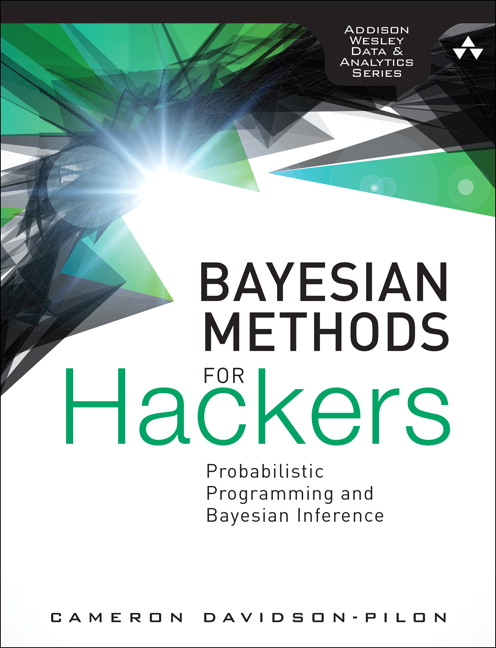This book includes major topics like Bayesian inference, loss functions, Bayesian machine learning, and Priors, which are suitable for non-statisticians with working knowledge of Python.

Bayesian interference deals with uncertainty understanding, and data scientists require learning this, where they need to model regularly. Machine learning engineers need this to learn for understanding the uncertainty related to the predictions for model delivery.

Bayesian methods are quite abstract and difficult to understand. Hence, this book is for programmers with knowledge of Python. This book explains the concepts in a simple way for non-statisticians. These are coded examples, throughout, and the Github repository, where the chapters are hosted has a large selection of notebooks. It is an excellent hands-on introduction subject book.

Timothy C. Urdan’s Statistics in Plain English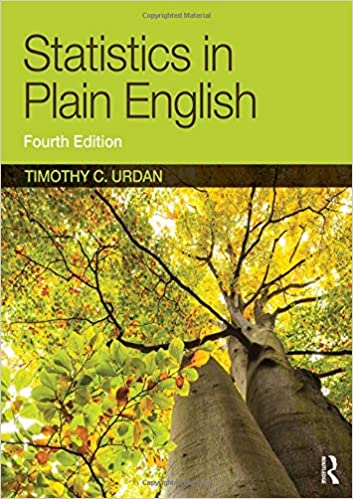This book includes topics like regression, distribution, factor analysis, and probability, which are suitable for non-statisticians having any level of programming experience.

It covers general statistical techniques. It is written in a direct manner, covering a wide range of depth of statistical concepts, in a simple understandable way. Written for students studying non-mathematics related courses, this book covers enough theory for understanding the techniques. It is ideal for learners without mathematics background entering data science.

Bradley Efron and Trevor Hastie’s Computer Age Statistical Inference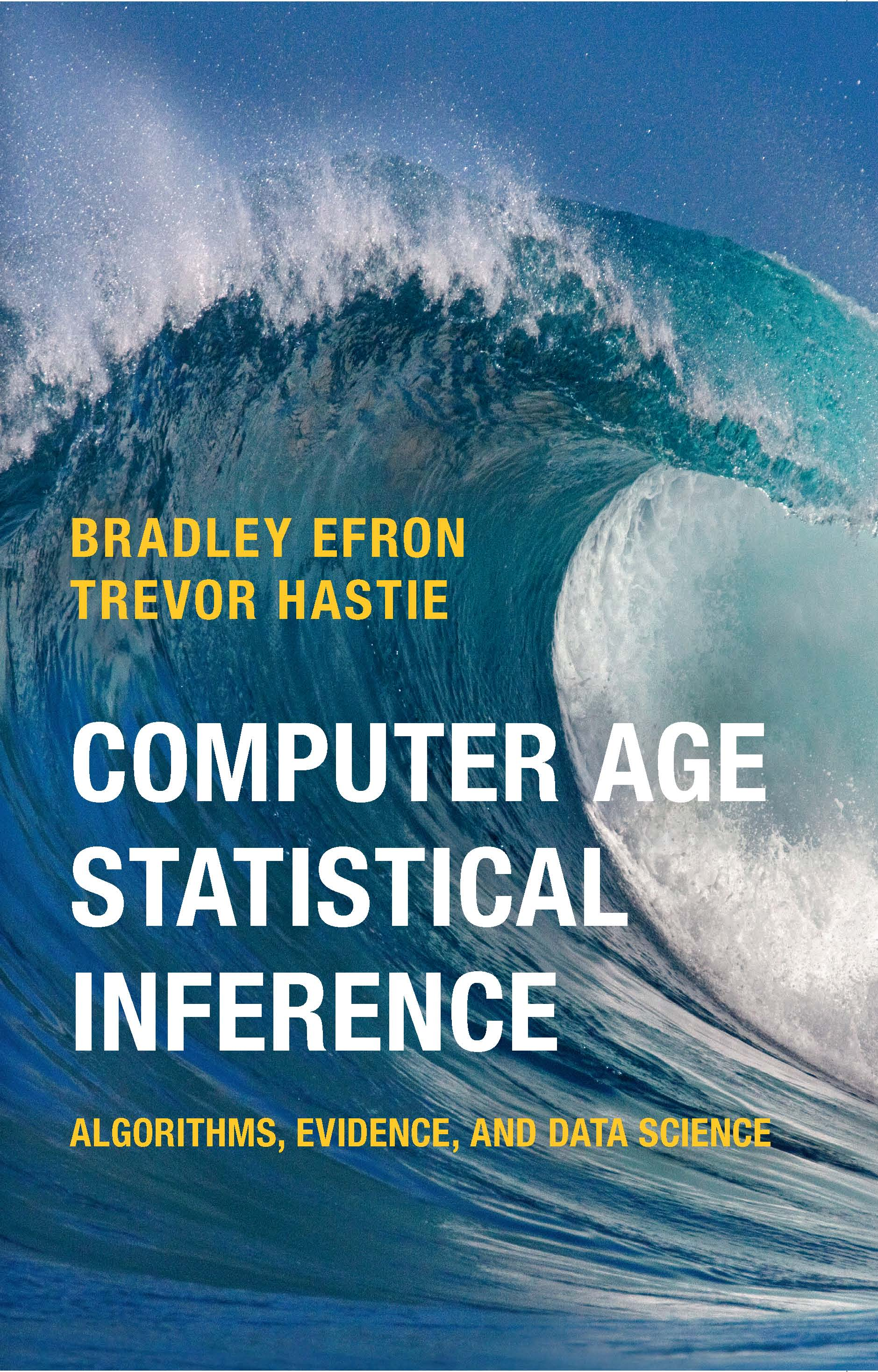This book includes Bayesian and Frequentist inference, Large scale hypothesis testing, Machine learning, and Deep Learning, which is suitable for learners with basic statistic knowledge and statistical notation, no coding/programming knowledge required.

The book has included the theory of popular machine learning algorithms, which are used by data scientists at present. It introduces both Bayesian and Frequentist statistical inference methodologies.

In the latter half of the book, learners learn machine learning algorithms, from some of the best content of education available. Concepts are explained in-depth, giving practical examples, of spam data like complex ideas. Learners with basic statistics in data analysis, who are familiar with statistical notation are most suited.

These are the books from my viewpoint, however, opinions may vary.